PS: 这里只是课程相关笔记

# 1. Basic Idea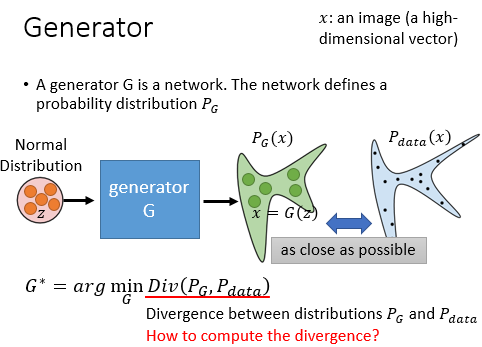GAN 的目的是找到一个 Generator 使得生成的数据的分布 $P_G(x)$ 和真实数据的分布 $P_{data}(x)$ 的散度尽可能解决。但是我们不知道  $P_{data}(x)$ 和 $P_G(x)$ 的分布。。。

GAN 通过 Discriminator 来衡量上面两个 distribution 的 divergence sampled $P_{data}$ and $P_G$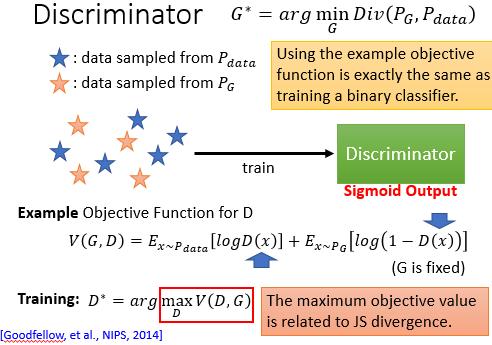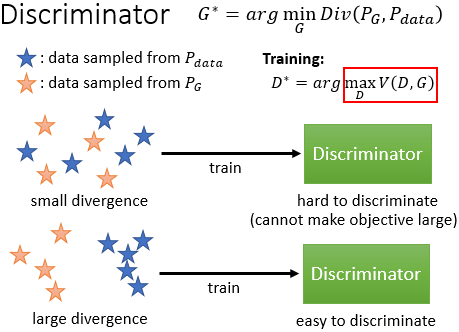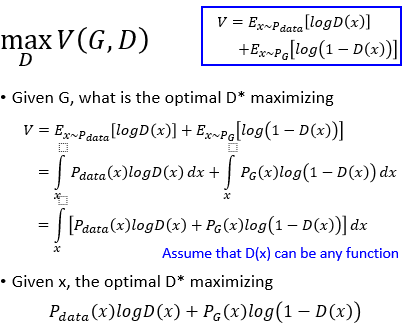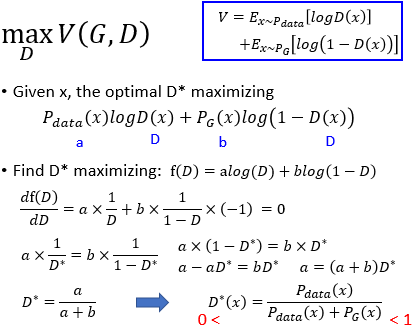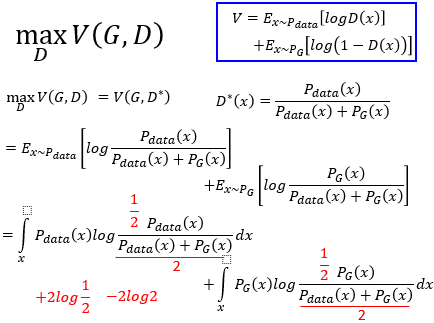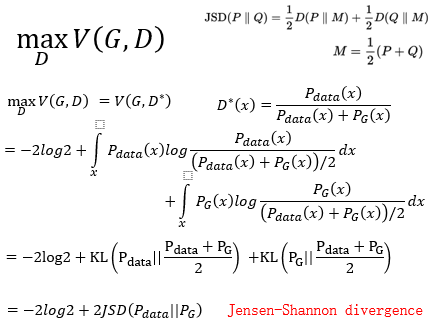\begin{equation}
\label{a}
\mathop{min}\limits_{G} \mathop{max}\limits_{D} V(D, G) \\
V(D, G) = E_{x \sim p_{data}(x)} [logD(x)] + E_{z \sim p_{z}(z)} [log(1-D(G(z))]
\end{equation}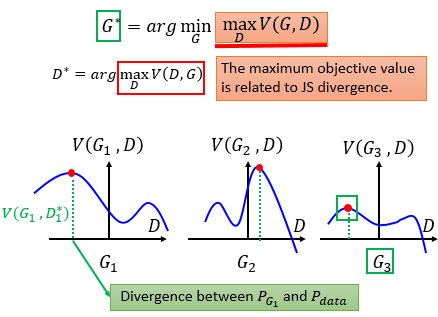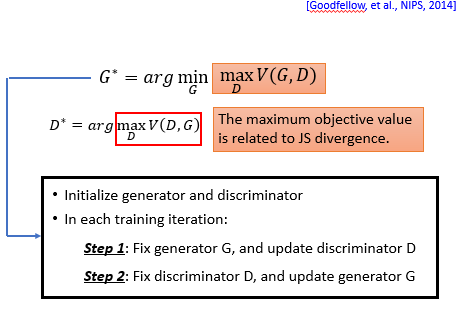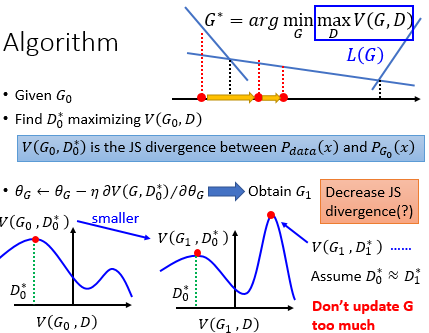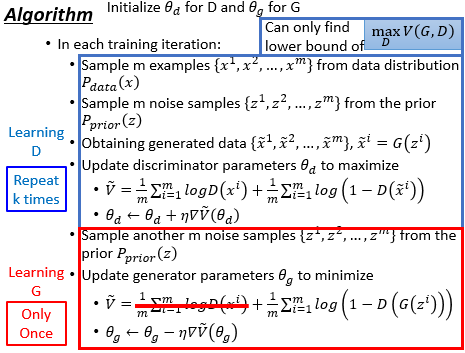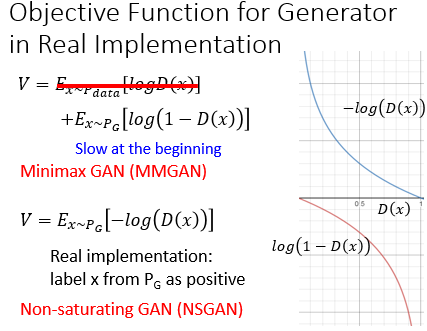# 2. Problem

## 2.1 Model Collapse

Model Collapse 现象是，你训练时时 real data 的 distribution 是比较大的，但是你 generate 出来的 example 的 distribution 是非常小的，举例来说，如果你训练次数较多时，生成的数据可能是下图这样的，会生成很多相似的图片：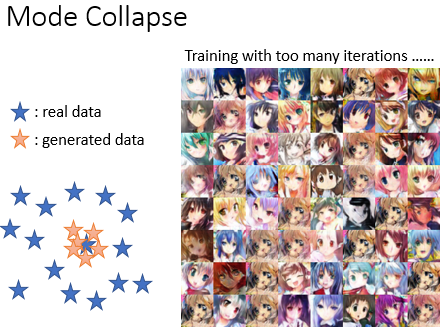## 2.2 Model Dropping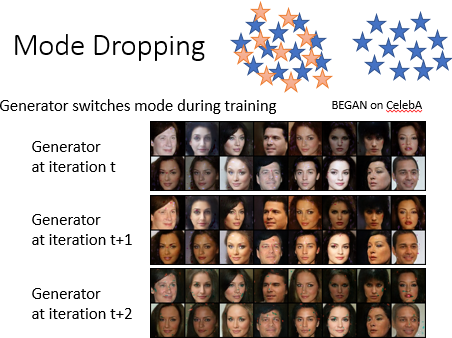posted @ 2020-05-24 12:34  xuanyuyt  阅读(63)  评论(0编辑  收藏